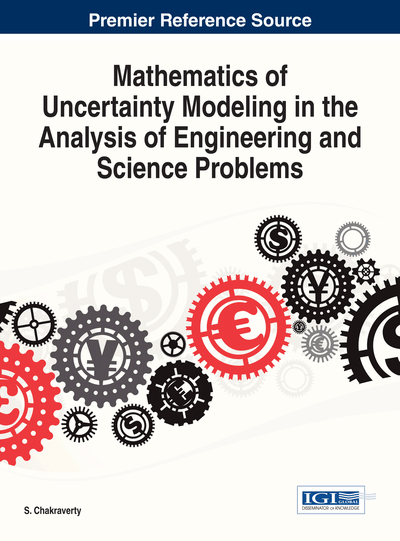# Reaction-Diffusion Problems with Stochastic Parameters Using the Generalized Stochastic Finite Difference Method

Marcin Kamiński (Technical University of Łódź, Poland) and Rafał Leszek Ossowski (Technical University of Łódź, Poland)
DOI: 10.4018/978-1-4666-4991-0.ch010

## Abstract

The main aim of this work is to demonstrate the new stochastic discrete computational methodology consisting of the generalized stochastic perturbation technique and of the classical Finite Difference Method for the regular grids to model reaction-diffusion problems with random time series. The generalized stochastic perturbation approach is based on the given order Taylor expansion of all random variables. A numerical algorithm is implemented here using the Direct Differentiation Method of the reaction-diffusion equation with respect to the height of a channel in 1D problem; further symbolic determination of the probabilistic moments and characteristics is completed by the computer algebra system MAPLE, v. 14. Computational illustration attached proves that it is possible to determine using this approach up to the fourth order probabilistic moments and coefficients as well as to consider time series with random coefficients for any dispersion of the input variables. Stochastic fluctuations of the input uncertainty source are defined here as the power time series with Gaussian random coefficients having given first two moments.
Chapter Preview
Top

## 1. Introduction

The Finite Difference Method (FDM) (Collatz, 1960; Forsythe & Wasow, 1959) plays an important role in computational engineering in all these cases, where the additional differential equations (ordinary or partial) may be solved straightforwardly – i.e. in the heat transfer (Minkowycz, 1988), electromagnetics (Taflove, 1998) and geodynamics. The basic idea of FDM is well known and involves replacing the differential quotient of the partial derivatives in the considered differential equations by the differences operators of various orders depending upon the desired numerical accuracy of the solution; this methodology with its numerous examples has been widely described by LeVeque (2007). So that, it seems to be natural that the FDM is extended towards its new stochastic versions for some real systems with random or stochastic parameters modeled before using traditional FDM in deterministic cases. One of such extension methods is the generalized stochastic perturbation-based technique, where the Taylor series expansions of all random quantities inserted into the given governing equation of the problem lead to the system of equilibrium equations of the ascending order (Kleiber & Hien, 1992). This method was employed before for different stochastic implementations for the Finite Element Method (Kleiber & Hien, 1992), Boundary Element Method as well as even Finite Difference Method, according to the second order second moment approach (Kamiński, 2001), but this implementation for the first time enables for

• 1.

Any order stochastic expansion,

• 2.

Any probability density function of the random input variable (Kamiński, 2007),

• 3.

Parametric study with respect to the perturbation parameter and coefficient of variation of the random input

• 4.

Analytical derivations of most of discrete hierarchical equations implemented in the computer algebra system MAPLE as well as

• 5.

Computational analysis with the stochastic processes.

The major difference in the comparison to the stochastic versions of a FEM and BEM is the necessity of double independent differentiation – with respect to the space variable (grid density) denoted by Δx as well as with respect to the chosen input random variable. Fortunately, because of an application of the symbolic calculus, this second differentiation is performed analytically for any available derivatives orders but in the case of a general computer program these derivatives must be implemented into it in a form of the ready-to-use-formulas (or calculated in a similar manner thanks to the interoperability with the MAPLE environment). The remaining implementation issues are almost the same like for the SFEM and the SBEM, but in a further perspective a comparison with other stochastic methods like polynomial chaos expansions (Ghanem & Spanos, 1991) or Monte-Carlo simulations (Hurtado & Barbat, 1998) would be interesting.

The stochastic implementation of the Finite Difference Method is displayed and discussed here on the example of the reaction-diffusion differential equation, that and seems to be quite natural because of numerous sources of uncertainty and stochasticity in chemical reactions and processes; such a computational methodology may essentially influence reliability modeling in the area of chemical engineering. The other reason to deal with this equation comes from various traditional and modern biotechnological applications related to the reaction-diffusion problems (Schnakenberg, 1987), which makes them still attractive also for the mathematical extensive studies (Kunert, 2005; Prieto et al., 2011). Although the general computer program is written in the symbolic computing environment MAPLE, the algorithm has a general character and the unidirectional grid may be essentially condensed without any larger programming issues; a formation and a solution of the ascending order hierarchical equations typical for the perturbation-based methodology will remain the same. This methodology will be further extended towards 2 and 3-dimensional applications, also for transient and nonlinear problems with both random and stochastic coefficients.

## Complete Chapter List

Search this Book:
Reset